# Blog

## 5000+ Members

### MEETUPS

LEARN, CONNECT, SHARE

### JOB POSTINGS

INDEED POSTINGS

Browse through the latest deep learning, ai, machine learning postings from Indeed for the GTA.

### CONTACT

CONNECT WITH US

Are you looking to sponsor space, be a speaker, or volunteer, feel free to give us a shout.

# What is My Data Worth?

People give massive amounts of their personal data to companies every day and
these data are used to generate tremendous business values. Some
economists
and
politicians
argue that people should be paid for their contributions—but the
million-dollar question is: by how much?

AISTATS and
VLDB papers that attempt to answer this
question in the machine learning context. This is joint work with David Dao,
Boxin Wang, Frances Ann Hubis, Nezihe Merve Gurel, Nick Hynes, Bo Li, Ce Zhang,
Costas J. Spanos, and Dawn Song, as well as a collaborative effort between UC
can be found here.

# What are the existing approaches to data valuation?

Various ad-hoc data valuation schemes have been studied in the literature and
some of them have been deployed in the existing data marketplaces. From a
practitioner’s point of view, they can be grouped into three categories:

• Query-based pricing attaches values to user-initiated queries. One simple
example is to set the price based on the number of queries allowed during a
time window. Other more sophisticated
examples
attempt to
adjust the price to some specific criteria, such as arbitrage avoidance.

• Data attribute-based
pricing

constructs a price model that takes into account various parameters, such as
data age, credibility, potential benefits, etc. The model is trained to match
market prices released in public registries.

• Auction-based
pricing
designs
auctions that dynamically set the price based on bids offered by buyers and
sellers.

However, existing data valuation schemes do not take into account the following
important desiderata:

• Task-specificness: The value of data depends on the task it helps to
fulfill. For instance, if Alice’s medical record indicates that she has
disease A, then her data will be more useful to predict disease A as opposed
to other diseases.

• Fairness: The quality of data from different sources varies dramatically.
performance via data poisoning attacks. Hence, the data value should reflect
the efficacy of data by assigning high values to data which can notably
improve the model’s performance.

• Efficiency: Practical machine learning tasks may involve thousands or
billions of data contributors; thus, data valuation techniques should be
capable of scaling up.

With the desiderata above, we now discuss a principled notion of data value and
computationally efficient algorithms for data valuation.

# What would be a good notion for data value?

Due to the task-specific nature of data value, it should depend on the utility
of the machine learning model trained on the data. Suppose the machine learning
model generates a specific amount of profit. Then, we can reduce the data
valuation problem to a profit allocation problem, which splits the total
utility of the machine learning model between different data sources. Indeed,
it is a well-studied problem in cooperative game theory to fairly allocate
profits created by collective efforts. The most prominent profit allocation
scheme is the Shapley value. The Shapley value attaches a real-value number to
each player in the game to indicate the relative importance of their
contributions. Specifically, for \$N\$ players, the Shapley value of the player
\$i\$ (\$iin I={1,ldots,N}\$) is defined as

s_i = sum_{Ssubseteq Isetminus{i}} frac{1}{N{N-1choose |S|}}[U(Scup {i})-U(S)]

where \$U(S)\$ is the utility function that evaluates the worth of the player
subset S. In the definition above, the difference in the bracket measures how
much the payoff increases when player \$i\$ is added to a particular subset \$S\$;
thus, the Shapley value measures the average contribution of player \$i\$ to
every possible group of other players in the game.

Relating these game theoretic concepts to the problem of data valuation, one
can think of the players as training data sources, and accordingly, the utility
function \$U(S)\$ as a performance measure of the model trained on the subset S
of training data. Thus, the Shapley value can be used to determine the value of
each data source. The Shapley value is appealing because it is the only
profit allocation scheme that satisfies the following properties:

• Group rationality: the total utility of the machine learning model is
completely split between different data sources, i.e., \$sum_{i=1}^N s_i =
U(I)\$. This is a natural requirement because data contributors would expect
the total benefit to be fully distributed.

• Fairness: Two data sources that have identical contributions to the model
utility should have the same value; moreover, data sources with zero
contributions to all subsets of the dataset should not receive any payoff.

• Additivity: The values under multiple utilities add up to the value under
a utility that is the sum of all these utilities. This property generalizes
the data valuation for a single task to multiple tasks. Specifically, if each
task is associated with a utility function as the performance measure, with
the additivity property, we can calculate the multi-task data value by simply
computing the Shapley value with respect to the aggregated utility function.

Because the Shapley value uniquely satisfies the aforementioned properties and
naturally leads to a payoff scheme dependent on the underlying task, we employ
the Shapley value as a data value notion. While the outlined concept appears
plausible, it has some fundamental challenges: computing the Shapley value, in
general, requires evaluating the utility function for an exponential number of
times; even worse, evaluating the utility function means re-training the model
in the machine learning context. This is clearly intractable even for a small
dataset. Interestingly, by focusing on the machine learning context, some
opportunities arise to address the scalability challenge. Next, we show that
for the K-nearest neighbors (KNN) classification, one can obviate the need to
re-train models and compute the Shapley value in quasi-linear time—an
exponential improvement in computational efficiency!

# Efficient algorithms for KNN

To understand why KNN is amenable to efficient data valuation, we consider
\$K=1\$ and investigate the following simple utility function defined for 1NN:
\$U(S)=1\$ if the label of a test point is correctly predicted by its nearest
neighbor in \$S\$ and \$0\$ otherwise. For a given test point, the utility of a set
is completely determined by the nearest neighbor in this set to the test point.
Thus, the contribution of the point \$i\$ to a subset \$S\$ is zero if the nearest
neighbor in S is closer to the test point than \$i\$. When we re-examine the
Shapley value, we observe that for many \$S\$, \$U(Scup{i})-U(S)=0\$.
Figure 1 illustrates an example of such an \$S\$. This simple example shows the
computational requirement of the Shapley value can be significantly reduced for
KNN.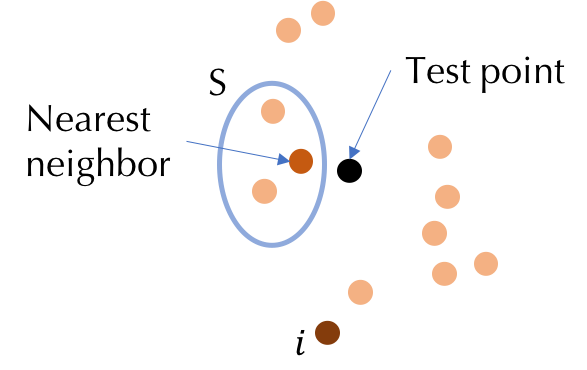Figure 1: Illustration of why KNN is amenable to efficient Shapley value
computation.

For a given test point \$(x_text{test},y_text{test})\$, we let \$alpha_k(S)\$
denote the \$k\$th nearest neighbor in \$S\$ to the test point. Consider the
following utility function that measures the likelihood of predicting the right
label of a particular test point for KNN:

U(S) = frac{1}{K}sum_{k=1}^{min{K,|S|}}mathbb{1}[y_{alpha_k(S)}=y_text{test}]

Now assume that the training data is sorted according to their similarity to
the test point. We develop a simple recursive algorithm to compute the Shapley
value of all training points from the furthest neighbor of the test point to
the nearest one. Let \$mathbb{I}[cdot]\$ represent the indicator function.
Then, the algorithm proceeds as follows:

%

This algorithm can be extended to the case where the utility is defined as the
likelihood of predicting the right labels for multiple test points. With the
additivity property, the Shapley value for multiple test points is the sum of
the Shapley value for every test point. The computational complexity is
\$mathcal{O}(Nlog NN_text{test})\$ for \$N\$ training points and \$N_text{test}\$
test points—this is simply the complexity of a sorting algorithm!

We can also develop a similar recursive algorithm to compute the Shapley value
for KNN regression. Moreover, in some applications, such as document retrieval,
test points could arrive sequentially and the value of each training point
needs to be updated and accumulated on the fly, which makes it impossible to
complete sorting offline. However, sorting a large dataset with a high
dimension in an online manner will be expensive. To address the scalability
challenge in the online setting, we develop an approximation algorithm to
compute the Shapley value for KNN with improved efficiency. The efficiency
boost is achieved by utilizing the locality-sensitive hashing to circumvent the
need of sorting. More details of these extensions can be found in our
paper
.

# Improving the efficiency for other ML models

The Shapley value for KNN is efficient due to the special locality structure of
KNN. For general machine learning models, the exact computation of the Shapley
value is inevitably slower. To address this challenge, prior work often resorts
to Monte Carlo-based approximation algorithms. The central idea behind these
approximation algorithms is to treat the Shapley value of a training point as
its expected contribution to a random subset and use the sample average to
approximate the expectation. By the definition of the Shapley value, the random
set has size \$0\$ to \$N-1\$ with equal probability (corresponding to the \$1/N\$
factor) and is also equally likely to be any subset of a given size
(corresponding to the \$1/{N-1choose |S|}\$ factor). In practice, one can
implement an equivalent sampler by drawing a random permutation of the training
set. Then, the approximation algorithm proceeds by computing the marginal
utility of a point to the points preceding it and averaging the marginal
utilities across different permutations. This was the state-of-the-art method
to estimate the Shapley value for general utility functions (referred to as the
baseline approximation later). To assess the performance of an approximation
algorithm, we can look at the number of utility evaluations needed to achieve
some guarantees of the approximation error. Using Hoeffding’s bound, it can be
proved that the baseline approximation algorithm above needs
\$mathcal{O}(N^2log N)\$ utility evaluations so that the squared error between the estimated and
the ground truth Shapley value is bounded with high probability. Can we reduce
the number of utility evaluations while maintaining the same approximation
error guarantee?

We developed an approximation algorithm that requires only sublinear
\$mathcal{O}(sqrt{N}(log N)^2)\$ utility evaluations by utilizing the
information sharing between different random samples. The key idea is that if a
data point has a high value, it tends to boost the utility of all subsets
containing it. This inspires us to draw some random subsets and record the
presence of each training point in these randomly selected subsets. Denoting
the appearance of the \$i\$th and \$j\$th training data by \$beta_i\$ and \$beta_j\$.
We can smartly design the distribution of the random subsets so that the
expectation of \$(beta_i-beta_j)U(beta_1,ldots,beta_N)\$ is equal to
\$s_i-s_j\$. We can pick an anchor point, say, \$s_1\$, and use the sample average
of \$(beta_i-beta_1)U(beta_1,ldots,beta_N)\$ for all \$i=2,ldots,N\$ to
estimate the Shapley value difference from all other training points to \$s_1\$.
Then, we can simply perform a few more utility evaluations to estimate \$s_1\$,
which allows us to recover the Shapley value of all other points. More details
of this algorithm can be found in our
paper
. Since this algorithm computes the
Shapley value by simply examining the utility of groups of data, we will refer
to this algorithm as the group testing-based approximation hereinafter. Our
paper also discusses even more efficient ways to estimate the Shapley value
when new assumptions can be made, such as the sparsity of the Shapley values
and the stability of the underlying learning algorithm.

# Experiments

First, we demonstrate the efficiency of the proposed method to compute the
exact Shapley value for KNN. We benchmark the runtime using a 2.6 GHZ Intel
Core i7 CPU and compare the exact algorithm with the baseline Monte-Carlo
approximation. Figure 2(a) shows the Monte-Carlo estimate of the Shapley value
for each training point converges to the result of the exact algorithm with
enough simulations, thus indicating the correctness of our exact algorithm.
More importantly, the exact algorithm is several orders of magnitude faster
than the baseline approximation as shown in Figure 2(b) .

<!–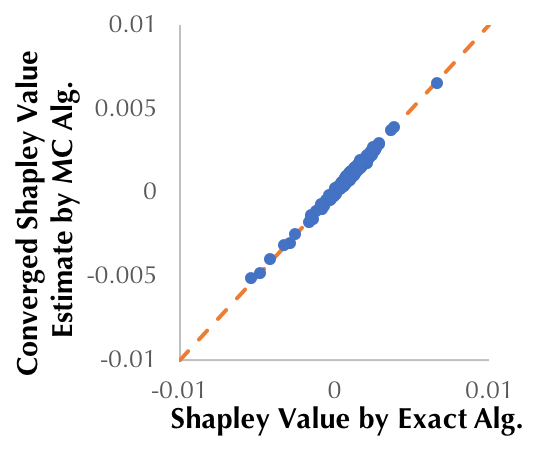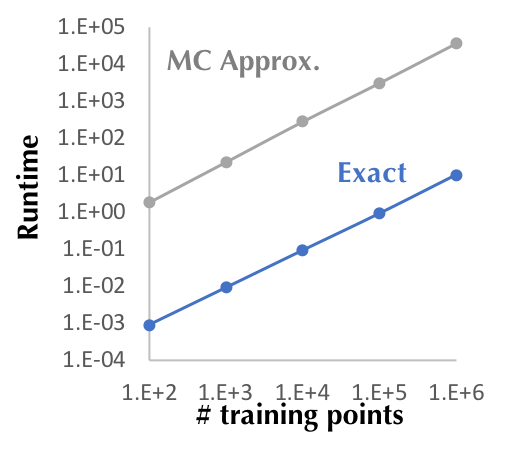–>Figure 2: (a) The Shapley value produced by our proposed exact approach and the
baseline Monte-Carlo approximation algorithm for the KNN classifier constructed
with 1000 randomly selected training points from MNIST. (b) Runtime comparison
of the two approaches as the training size increases.

With the proposed algorithm, for the first time, we can compute data values for
a practically large database. Figure 3 illustrates the result of a large-scale
experiment using the KNN Shapley value. We take 1.5 million images with
pre-calculated features and labels from Yahoo Flickr Creative Commons 100
Million (YFCC100m) dataset. We observe that the KNN Shapley value is
intuitive—the top-valued images are semantically correlated with the
corresponding test image. This experiment takes only a few seconds per test
image on a single CPU and can be parallelized for a large test set.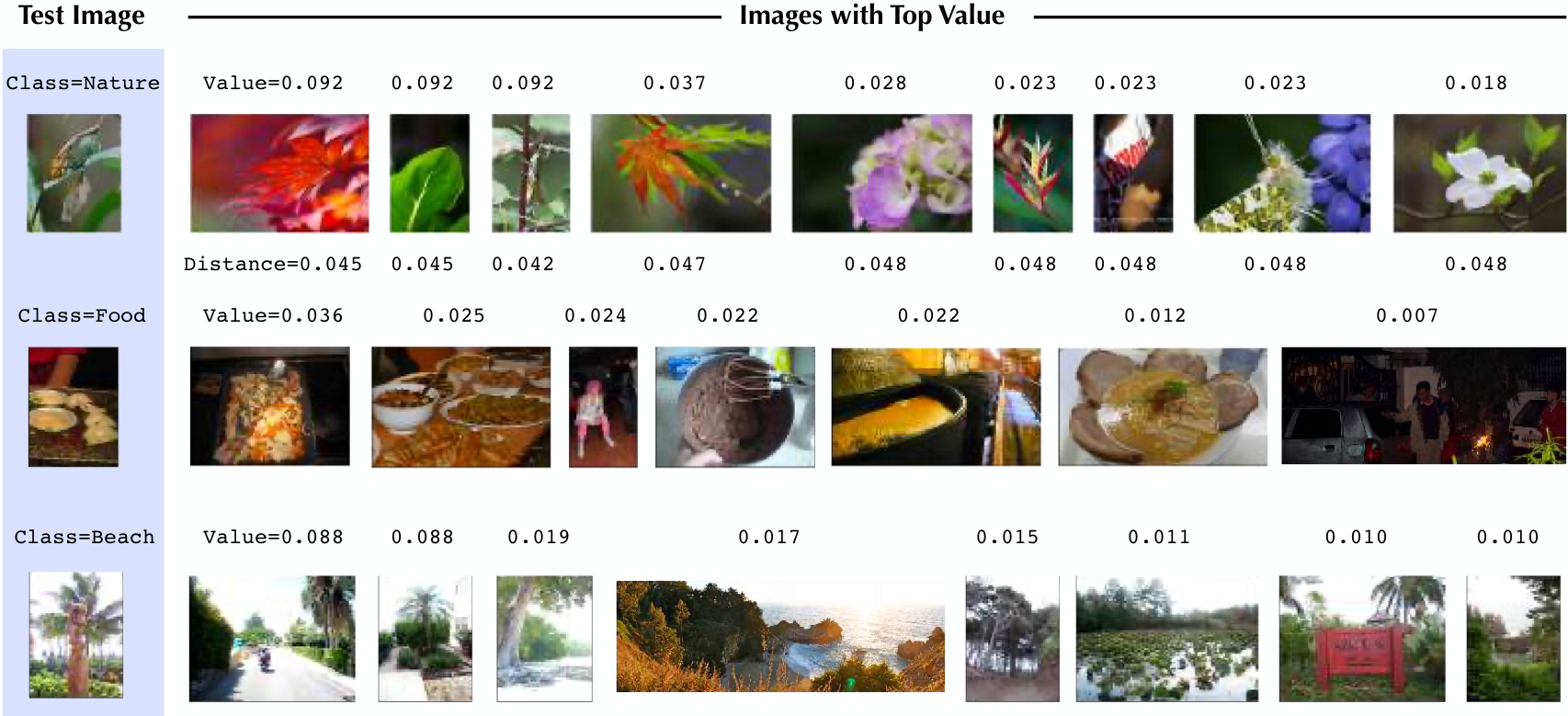Figure 3: Data valuation using KNN classifiers (K = 10) on 1.5 million images
(all images with pre-calculated deep feature representations in the Yahoo100M
dataset).

Similarly, Figure 4(a) demonstrates the accuracy of our proposed group
testing-based approximation and Figure 4(b) shows that the group testing-based
approximation outperforms the baseline approximation by several orders of
magnitude for a large number of data points.

<!–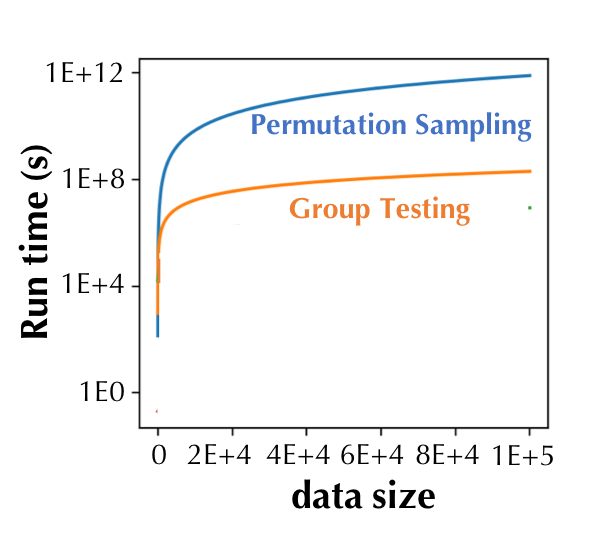–>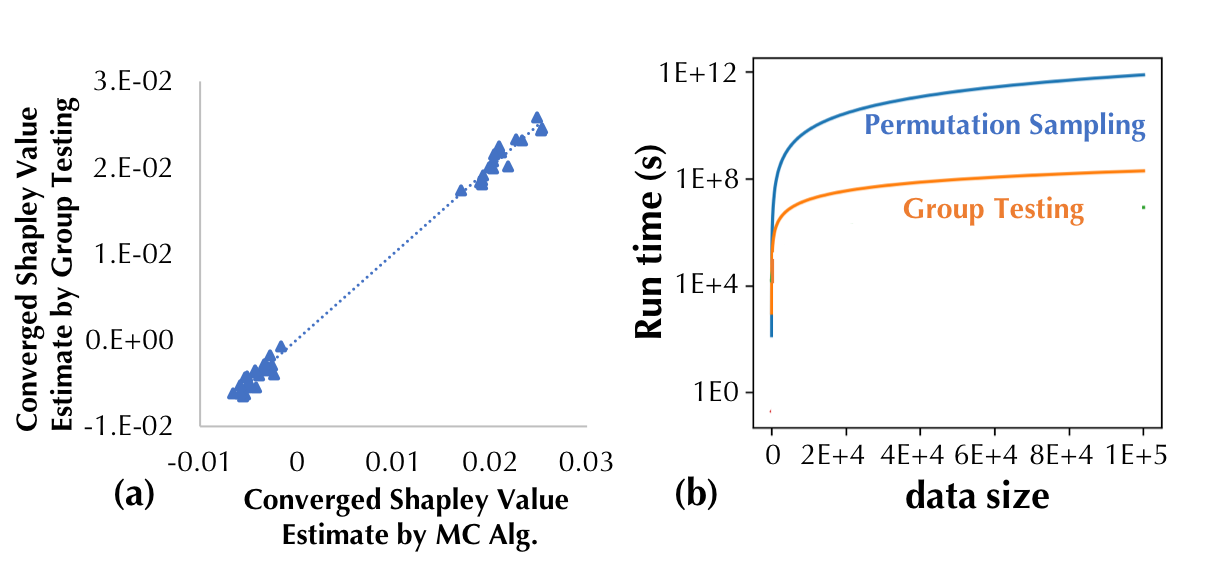Figure 4: The Shapley value produced by our proposed group testing-based
approximation and the baseline approximation algorithm for a logistic
regression classifier trained on the Iris dataset. (b) Runtime comparison of
the two approaches.

We also perform experiments to demonstrate the utility of the Shapley value
beyond data marketplace applications. Since the Shapley value tells us how
useful a data point is for a machine learning task, we can use it to identify
the low-quality or even adversarial data points in the training set. As a
simple example, we artificially create a training set with half of the data
directly from MNIST and the other half perturbed with random noise. In
Figure 5, we compare the Shapley value between normal and noisy data as the
noise ratio becomes higher. The figure shows that the Shapley value can be used
to effectively detect noisy training data.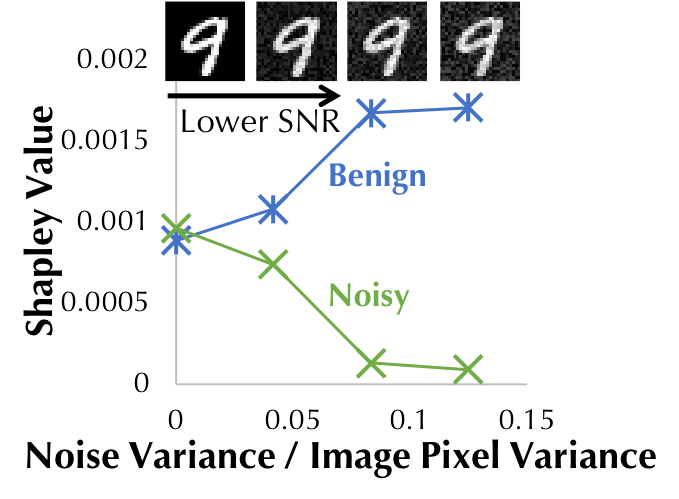Figure 5: The Shapley value of normal and noisy training data as the noise
magnitude becomes higher.

The Shapley value can also be used to understand adversarial training, which is
an effective method to improve the adversarial robustness of a model by
introducing adversarial examples to the training dataset. In practice, we
measure the robustness in terms of the test accuracy on a dataset containing
dataset become more valuable as more adversarial examples are added into the
test dataset. Based on the MNIST, we construct a training dataset that contains
both benign and adversarial examples and synthesize test datasets with
different adversarial-benign mixing ratios. Two popular attack algorithms,
namely, the fast gradient sign method (FGSM)
and the iterative attack (CW) are used to
generate adversarial examples. Figure 6(a) and (b) compare the average Shapley
value for adversarial examples and for benign examples in the training dataset.
The negative test loss for logistic regression is used as the utility function.
We see that the Shapley value of adversarial examples increases as the test
data becomes more adversarial; in contrast, the Shapley value of benign
are more valuable if they are generated from the same attack algorithm during
test time.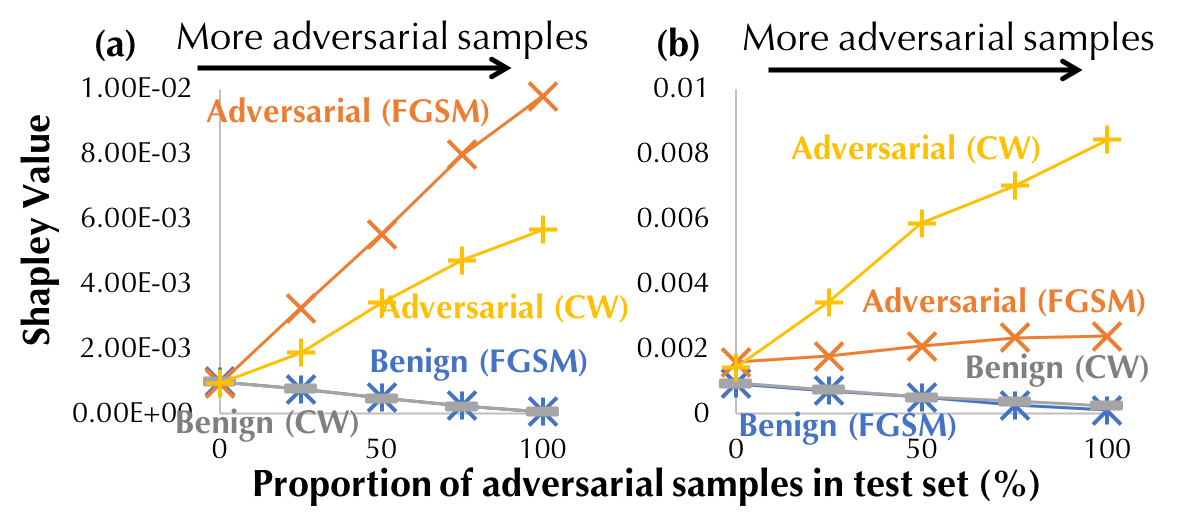Figure 6: Comparison of the Shapley value of benign and adversarial examples.
FGSM and CW are different attack algorithms used for generating adversarial
examples in the test dataset: (a) (resp. (b)) is trained on Benign+FGSM (resp.

# Conclusion

We hope that our approaches for data valuation provide the theoretical and
computational tools to facilitate data collection and dissemination in future
data marketplaces. Beyond data markets, the Shapley value is a versatile tool
for machine learning practitioners; for instance, it can be used for selecting
features or interpreting black-box model predictions. Our algorithms can also
be applied to mitigate the computational challenges in these important
applications.

# Plug yourself into AI and don't miss a beat

Toronto AI is a social and collaborative hub to unite AI innovators of Toronto and surrounding areas. We explore AI technologies in digital art and music, healthcare, marketing, fintech, vr, robotics and more. Toronto AI was founded by Dave MacDonald and Patrick O'Mara.